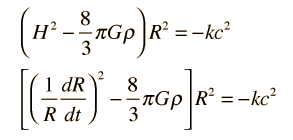# Mathematical Modeling of the Written Universe

## The Friedmann equation and the expanding universe

Convenient forms of Friedmann’s equation with which to examine the expansion time and temperature for a big bang model of the actual universe areSimple forms of Friedmann’s equations. See this link for source material. Material in italics was copy pasted almost verbatim (aka plagiarized) from the source. Thank you in advance HyperPhysics for not suing me.

Besides the density and gravitation constant G, the equation contains the Hubble parameter H, a scaling parameter R, and a factor k which is called the curvature parameter. The curvature parameter indicates whether the universe is open or closed. The above equations are highly simplified and do not specify the nature of the density ρ, nor do they include any particle interactions other than gravitational attraction. Such particle interactions, like collisions, could be specified in terms of pressure, so the model above is sometimes referred to as a “pressureless” universe. More detailed versions of the Friedman equation include such effects.

## The Chrichton Parameter

• If k = 0 then the density is equal to a critical value at which the amount of total crap written will expand forever at a decreasing rate. This is often referred to as the Martin-Koontz universe in recognition of their work in modeling it. This k = 0 condition can be used to express the critical density in terms of the present value of the Harlequin Romance parameter.
• For k > 0 the density is high enough that the King attraction from all writing will eventually stop the expansion of crap and it will collapse backward to a “big crunch”. This kind of written universe is described as being a closed written universe, or a King bound universe.
• For k < 0 the amount of terrible writing expands forever, there not being a sufficient total amount of writing for King attraction to stop the expansion.

Remember that in our written universe model the scaling parameter is hypothesized to be nearly infinite which suggests the probability of k < 0 is very high. Thus if this model holds we almost certainly live in a written universe in which the amount of terrible writing will continue to expand forever. This very article being a fine example of why that is most likely the case.

Written by